# Geometry

#### Coordinate Geometry - 2

Lines and Angles

Lines, Angles and Basics of Triangles:

Angles:

Angle is a figure formed when two rays share a common end point. These rays are called the sides while the common point is called the vertex of the angle.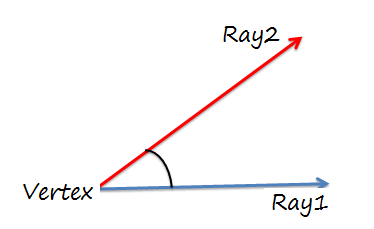The different types of angles are:

1. Acute Angle:- An angle whose measure is less than 900.
2. Right Angle:- An angle whose measure is equal to 900.
3. Obtuse Angle:- An angle whose measure is more than 900.
4. Complementary Angles:- Two angles whose sum is 900.
5. Supplementary Angles:- Two angles whose sum is 1800.

Example 1: What is the measure of an angle whose complement is 37.5% of its supplementary angle?

1. 240           2. 300            3. 360       4. 450

Solution: Let the angle be ‘θ’ . So, its complement is equal to 90 – θ and its supplement is equal to 180- θ

(90- θ) = 0.375 * (180- θ)

Or θ = 360

Example 2. The sum of two angles is 800. If one of the angles is one third of the complement of the other angle, then the measure of these angles is-

1. 50,750           2. 250, 550        3. 200,600       4. None of these

Solution: Let the two angles be α and β.

So, α + β = 800  and   α = 1/3 (90 – β)

Solving these equations we get α = 50 and β = 750

Important:

If a ray stands on a line , then the sum of two adjacent angles so formed is 1800 and they are called a linear pair of angles.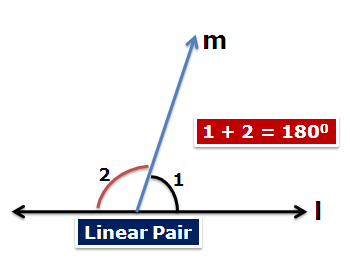Vertically Opposite Angles: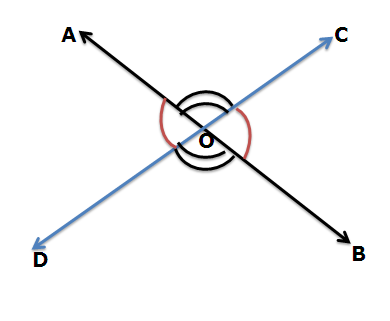If two lines intersect each other, then the vertically opposite angles are equal. Here AOC = BOD and also AOD = BOC

Example 3. In the given figure, AO is perpendicular to XY. BC is a straight line that intersects XY at point O. If a:b = 7:8 and e:d = 4:7 , then the value of d is?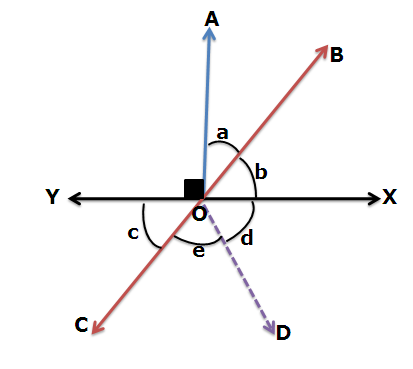1. 420           2. 640               3. 720              4. 840

Solution: XY is a straight line and angle AOY is a right angle, so angle AOX is also equal to 900.

So, a + b = 900.  Also, a:b = 7:8

Thus, a = 420      and b = 480

Now, b = c {Vertically opposite angles }

Angle COX = 1800 – 480 = 1320

Also, e:d = 4:7  and  e + d = 1320

So, e = 480     and   d = 840

Parallel Lines:

Two lines in a plane are parallel to each other if the distance between them is constant.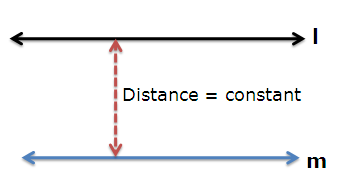Parallel Lines and a Transversal

Let two parallel lines l and m be intersected by a transversal ‘n’.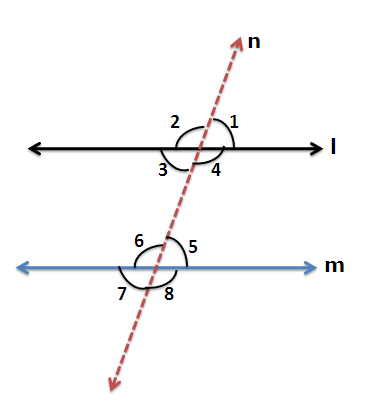Corresponding angles are equal: i.e. 1 = 5 , 2= 6 , 3 = 7 and 4 = 8.

Vertically opposite angles are equal, i.e. 1 = 3, 2 = 4 , 6 = 8 and 5=7

Alternate interior and exterior angles are equal, i.e.3 =5, 4=6,

2=8 and 1 =7

Co-interior Angles are Supplementary, i.e. 4+5 = 1800 and 3+6 = 1800

Note: If the angle bisectors of co-interior angles are joined, then they make a right angle with each other.

Example 4:  In the figure below, AB || DE. Also, CF is the angle bisector of angle BCD. What is the measure of DCF?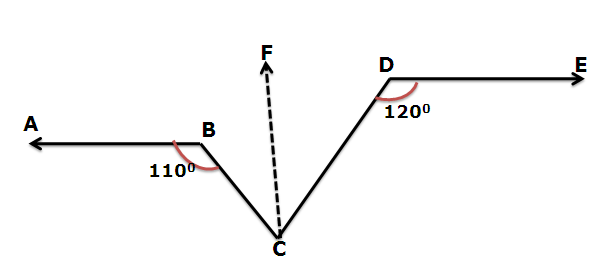Solution: Draw a line passing through C and parallel to AB and DE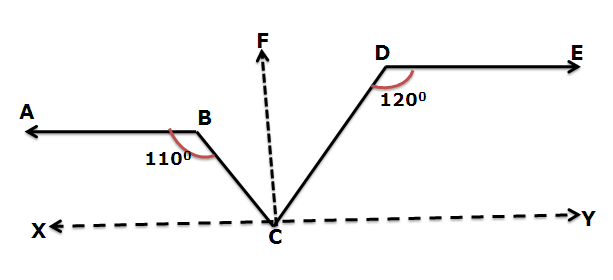AB || CX, so, ∠ XCB = 1800 - 1100 = 700

DE || CY, so, ∠ YCD= 1800 - 1200 = 600

So, ∠ BCD = 1800 – 700 -600 = 500

Now, CF is the angle bisector, so ∠ DCF = ½ * 50 = 250

Example 5.  In the given figure, AB || CD. What is the measure of angle BED?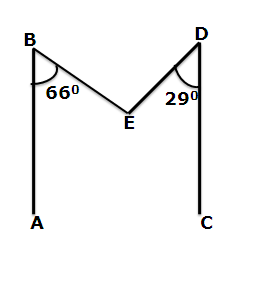Solution: Draw a line parallel to AB and CD passing through E.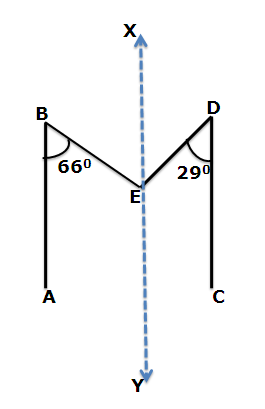∠ BEX = 660 { Alternate Interior Angles}

∠ DEX = 290 { Alternate Interior Angles}

So, ∠ BED = 660 + 290 = 950

Q6.  In the given figure AB || CD || EF. What is the value of x,y and z?Solution: ∠ PQR = 270 {Alternate Interior Angles}

∠PRQ = 1800 – 1320 = 480 { Linear Pair}

y = ∠QPR = 1800 – 270 – 480 = 1050 { Angle Sum Property of a Triangle}

x = 180 – ∠PQR = 1530{ Co-interior Angles are supplementary}

z = 180 – ∠PRQ= 1320 { Co-interior Angles are supplementary}

Triangles:

A triangle is a closed figure formed by joining three non collinear points. A triangle has three vertices, three angles and three sides.

Types of Triangles:

Based on Sides:

1. Scalene : It is a triangle whose all sides are of unequal lengths.

2. Isosceles : It is a triangle whose two sides are of equal lengths.

3. Equilateral :It is a triangle whose all sides are of equal lengths.

Based on Angles:

1. Acute Angled Triangle: It is a triangle in which all the three angles are acute i.e. each of them measure less than 900.

2. Right Angled triangle: It is a triangle in which exactly one angle is a right angle while the other two are acute angles.

3. Obtuse Angled Triangle: It is a triangle in which one angle is more than 900 i.e. obtuse while the other two angles are less than 900 i.e. acute.

Angle Sum Property:

It is a universal property that is applicable to every triangle that one can encounter. It states that the sum of all the angles of a triangle is 1800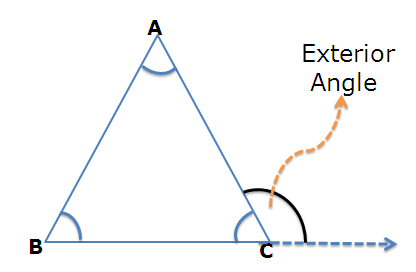i.e.   A + B + C = 1800

Also, exterior angle =  A  + B  i.e. exterior angle is equal to the sum of the  two opposite interior angles.

Triangle Inequality:

Now, this property is related to the sides and gives a relation between the three sides of any triangle. Again it is a universal property.

It says that the sum of two sides is always greater than the third side. i.e.

AB + BC > AC      or BC > AC - AB

AC+BC > AB        or AC > AB - BC

AB + AC > BC     or AB > BC – AC

It can also be said that any side is always greater than the difference of the other two sides.

Q7. The three sides of a triangle are 7, 15 and x units where x is an integer. What is the sum of all the possible values of x?

Solution: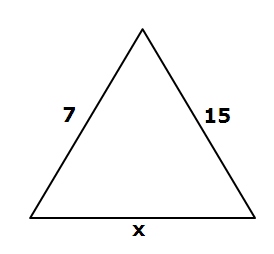Using the triangle inequality:

Either  x + 7 > 15              or 7 + 15 > x

x> 8            or x < 22

Thus, 8 < x < 22 . Now as x can take only integral values, so x = 9,10,11,…….., 21

Sum of all the possible values of x = 9 +10 + 11 + 12 + ……. + 21 = 195

Q8. If the sum of all the sides of a triangle is 8 units, where all the lengths are integers, then how many such triangles are possible?

1. 1          2. 2             3.3                    4. No such triangle can be formed.

Solution: Let the lengths of sides be a, b and c units respectively.

So, a + b + c = 8

Different possibilities are  (6,1,1) , (5,1,2) , (4,2,2), (4,3,1) and (3,3,2)

Out of these only (3,3,2) satisfies the triangle inequality. So only one triangle (3,3,2) is possible.

Q9.   In the given figure PQ =PR = RS. If ∠TPQ = 720   then,  the measure of  ∠QPR is-

1. 480               2. 720                3. 840                4. 960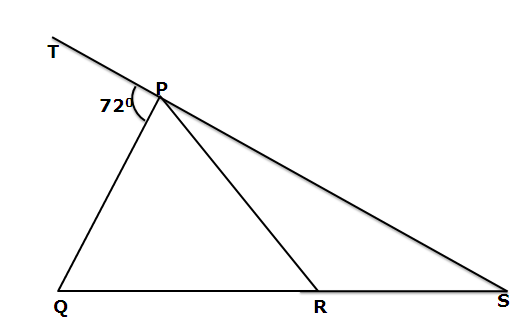Solution: PQ  = PR =  RS.

Let  PSR =  SPR = θ { Angles opposite to equal sides are equal}

QRP = PSR + SPR = 2θ { Exterior angle property }

PQR = QRP =2θ  { As PQ = PR }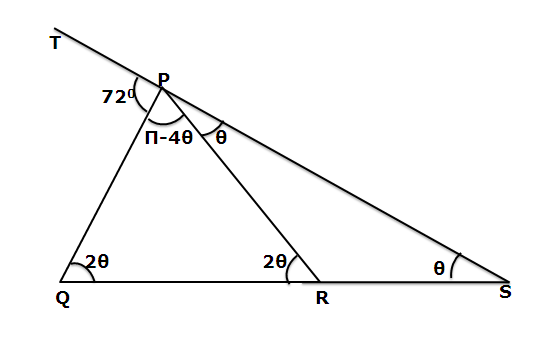As TPQ is an exterior angle, so

TPQ = PQR + PSQ

720 = 2θ + θ

Or θ = 240

Hence  ∠QPR = 180 – 4*24 = 840

Q10. In ΔABC, A = B = 700. Also BE and CD are the angle bisectors of B and C respectively. What is the value of x + y + z?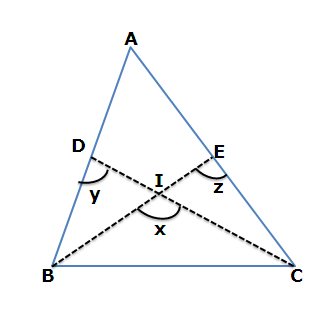Solution: As A = B = 700, so C = 400. Also, BE and CD are the angle bisectors.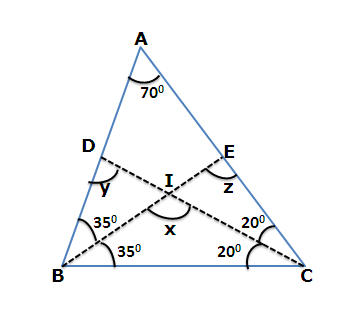In ΔBIC,  x = 1800 -350 -200 = 1250

In ΔBEC, z = 1800 – 350 -400 = 1050

In ΔBDC, y = 1800 – 700 – 200 = 900

Thus, x + y + z = 1250 + 1050 + 900 = 3200Q11. In a triangle ABC, AB = AC. BA is produced to D in such a manner that

AC = AD. The circular measure of BCD is -

1. ?/6             2. ?/3 3. 2?/3         4. ?/2

Solution: Given: AB = AC = AD. If ABC = ACB = θ, then DAC being the exterior angle of ΔABC,  DAC = θ+θ = 2θ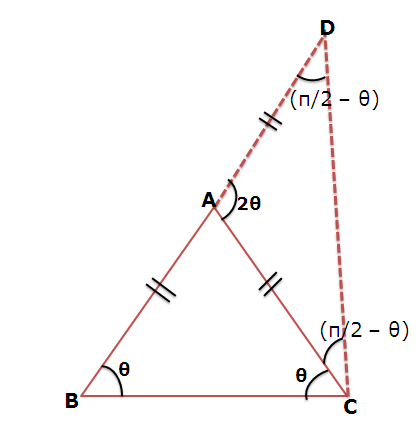∠BCD = BCA + ∠ACD = θ+ (?/2 – θ) = ?/2

Q12. In a ΔABC, AD is the median and AD = ½ BC. If ∠ BAD = 300, then measure of ∠ ACB is

1. 900     2. 450         3. 300        4. 600

Solution: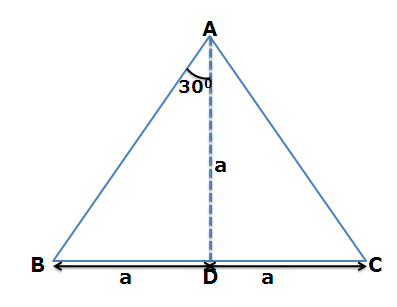Given that BD = AD = CD { As median divides the base into two equal parts}

In ΔABD, AD =BD , ∠ DBA = ∠DAB = 300 { Angles opp to equal sides }

Hence,  ∠ADC = 300 + 300 = 600 { Exterior angle}

Again in ΔADC, AD = CD ,hence ∠DAC = ∠DCA = θ

So, In ΔADC , θ + θ + 600 = 1800 { Angle sum property}

Or θ = 600

Therefore, ∠ ACB = 600

Q13. If G is the centroid of ΔABC and AG = BC, then ∠ BGC is –

1. 750       2. 450       3. 900           4. 600

Solution:  G is the point of intersection of medians of a triangle. Let BC = 2x, so AG = 2x.  Now, centroid divides the medians in ratio of 2:1. So If AG = 2x, then GD = x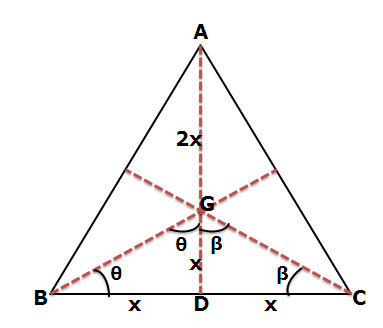In ΔBGD, BD = GD . So,  ∠DBG = ∠DGB = θ

Similarly in ΔGDC, GD = CD. So, ∠DCG = ∠DGC = β

Now in ΔBGC, ∠DBG + ∠BGC + ∠GCB = 1800

θ + θ + β + β = 1800

Or θ + β = 900

Or  ∠BGC = 900

Example 14 : In ΔABC , BCA= CDE = DEC = ABC, what is the measure of BAE?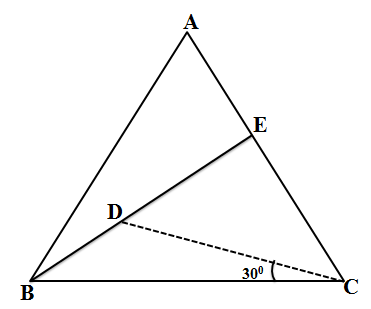Solution:  Let ∠DCE = α , So, ∠ABC = ∠BCA = ∠CDE = ∠ DEC = 30 + α

Consider  ΔDEC, Sum of all the angles must be 180

So, 30 + α + 30 + α + α = 180

3 α + 60 = 180   or  α = 400

So, ∠ABC = ∠BCA = ∠CDE = ∠ DEC = 70

∠BAE = 180 – 70 – 70 = 400

Example 15. In the given figure AB = BC = CD = ED = EF = FG = GH = HI = IJ = JK = AK. What is the value of θ ?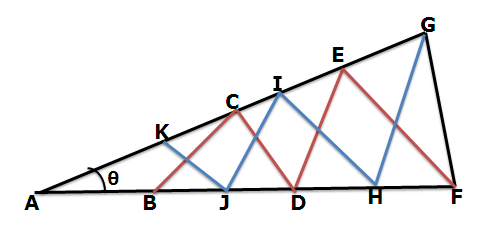Solution: If we look at the figure with all the blue lines removed, we get the following figure-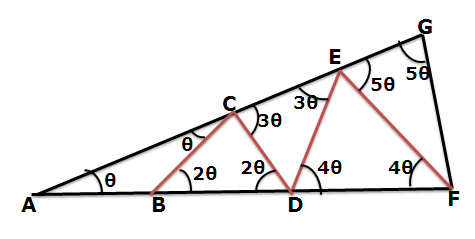BAC = BCA = θ

CBD = 2θ {Exterior Angle}

CDB = 2θ {As BC = CD}

DCE = 3θ{Exterior angle in ΔACD}

DCE = DEC { As CD = DE }

EDF = 4θ { Exterior angle in ΔAED}

EDF = EFD = 4θ { As ED = EF }

FEG = 5θ { Exterior angle in ΔAEF}

FGE = 5θ { As EF = GF}

Now if we look at the figure with all the red lines removed, the figure looks like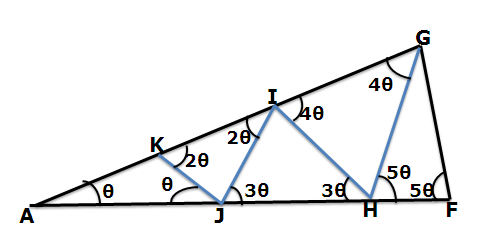All the angles are expressed in terms of ‘θ’ in the same manner as above.

Earlier we have seen FGE = FGA = 5θ

Also IGH = 4θ { Above figure}

So, FGH = θ

Now, in ΔGHF, 5θ + 5θ + θ = 1800 or

θ = 1800/11